# FLARE On Challenge (2015) #9

This challenge contains a Windows Portable Executable file in a very small size (4,608 bytes), typically, this kind of files is written in Assembly Lagrange. If you load this file into IDA, you may notice that the file is badly obfuscated and hard to analyze statically. But if you look at the Strings (Shift + F12), you may find some interesting code located at 0x00401000: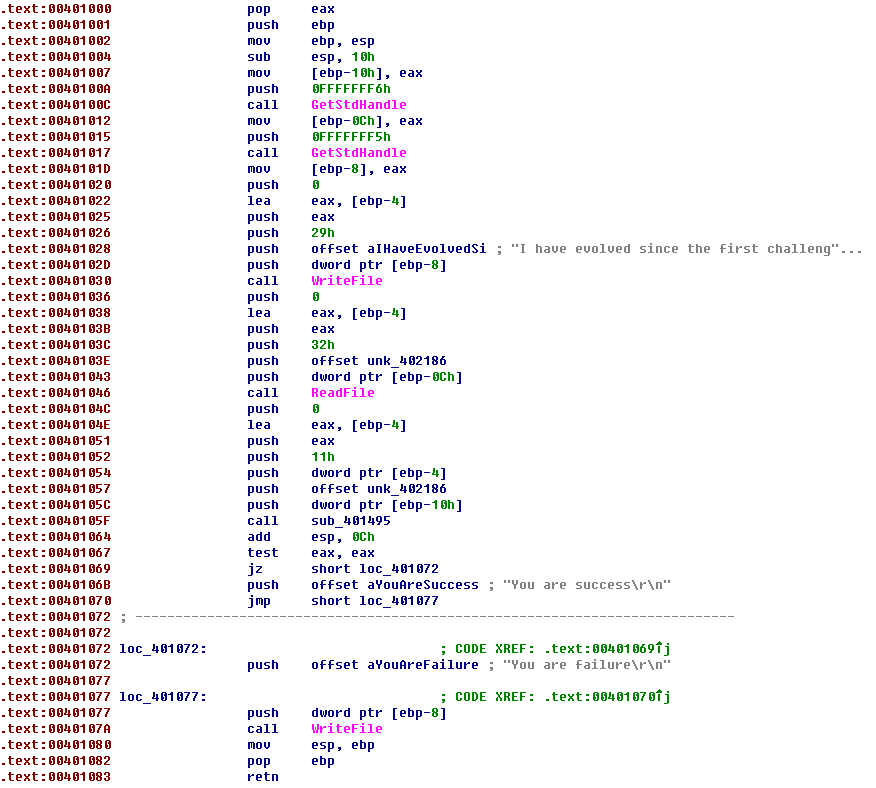Those code looks very similar to challenge #1 and challenge #2, so how about executing this program in a debugger and putting a breakpoint at 0x00401000? Unfortunately, the breakpoint here will not being hit. Eh… how about putting a breakpoint on the API it may calls like WriteFile() or ReadFile()? Badly, it seems cannot work either. But if you are not give up, you will finally find that a breakpoint on GetStdHandle() will eventually interrupt the execution of this program. That’s a good thing! Let’s execute this program step by step after hit the second GetStdHandle(). After several instructions, I am surprised that the program actually calls the API WriteFile():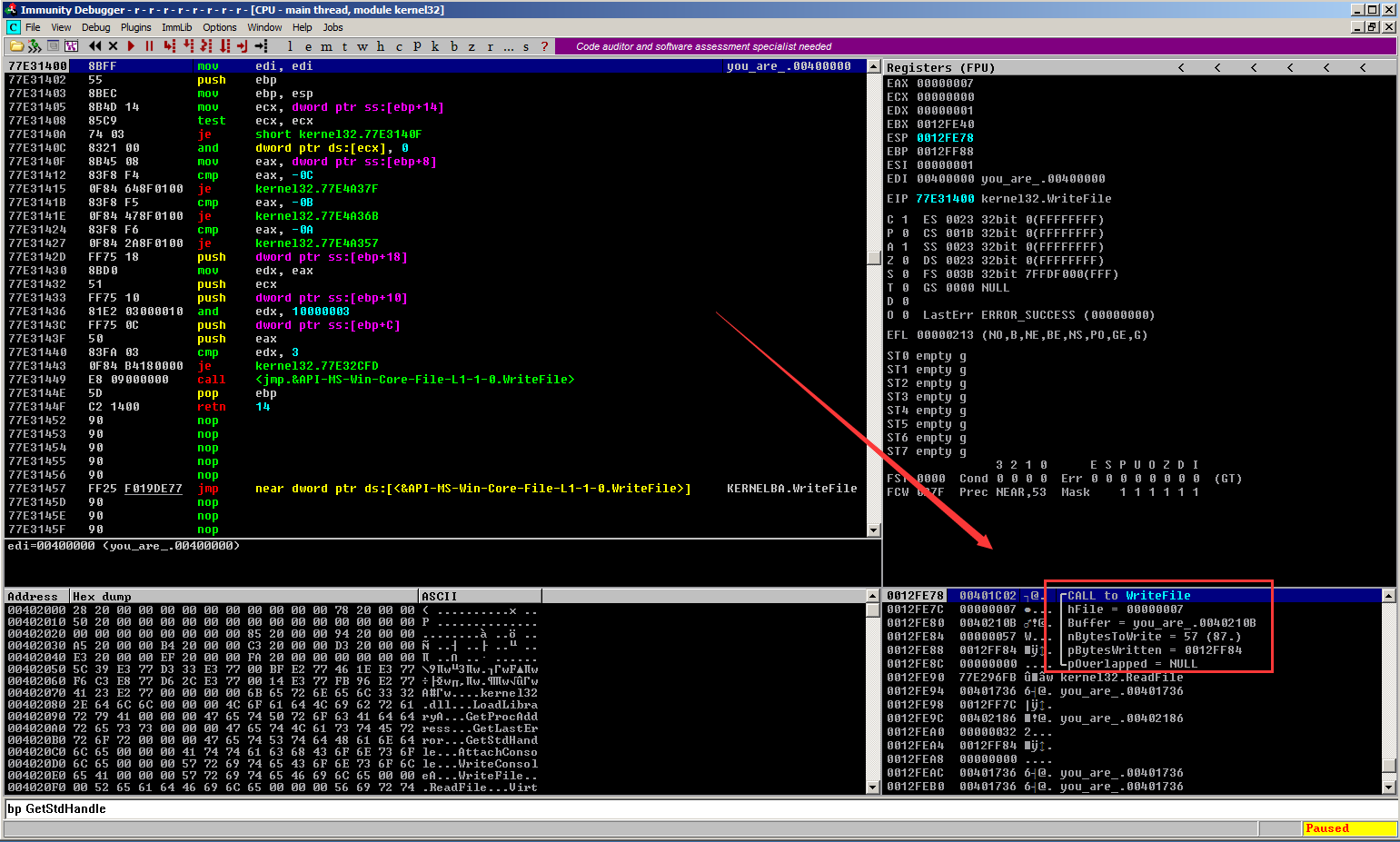So why our previous breakpoint on WriteFile() did not work? When I look at the breakpoints lists, I realized that it is because the debugger “play a joke” on me: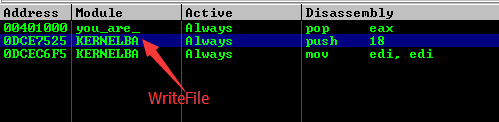The breakpoint here is set on the WriteFile() function from module Kernelbase.dll, however, the WriteFile() function being called at real time comes from module kernel32.dll: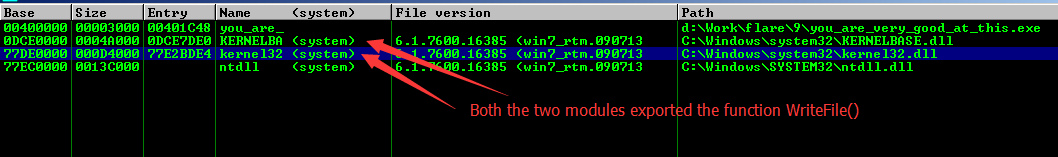Understand this, now we can put a breakpoint on the ReadFile() function in the kernel32.dll and it will hit as expected. After provides some fake input to the ReadFile() function, I continue executing this program on single steps. And finally, it comes to the interesting part: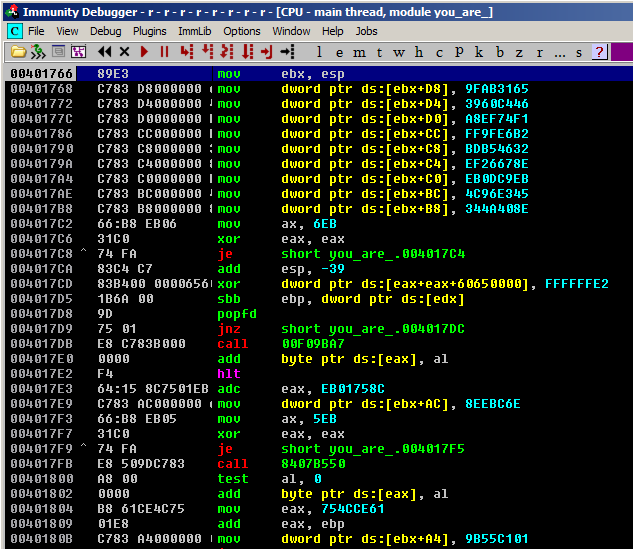If you execute the code from here step by step, you will find that although the code is badly obfuscated, the verification logic is easy to understand: firstly, it will initialize a table on the stack, and retrieve an index from this table, and then it will use this index to retrieve a second index from the same table. Next, it will use the second index to retrieve a byte from that table and XOR it with the relevant byte of our input data. After this, it will use the second index to retrieve another byte from that table and do a left rotation with the XOR result. And finally it will compare the rotate result with a third byte retrieved from that table.

If you cannot understand the algorithm clearly through my description, the following Python script may help:

```table  = '\xCC\xA9\x6D\x77\x0A\xF2\x4E\x58\xC1\x8A\x2F\x9F\xD8\x6C\x58\x43'
table += '\xF9\x1D\xC8\x92\x1F\x5C\xC3\x98\xC7\xF8\xD7\x03\xB0\x8A\x3C\xE7'
table += '\x1F\x1A\xCD\x02\x13\xF6\x07\x73\xF2\x33\xC8\xF0\xBA\xC2\xCC\xB8'
table += '\x80\xF0\x48\x77\xF9\x9A\x50\x55\xDC\x01\xE1\xEB\x16\x5A\x6B\xC6'
table += '\xC3\x0E\x8E\x27\x5D\xC3\xF4\x4E\x06\x42\xBC\xF2\x5D\x56\x30\x2E'
table += '\x19\x53\x53\x66\xD2\xF9\x03\xCF\x12\x06\x17\x1A\x0A\x0D\x02\x1E'
table += '\x16\x10\x1F\x09\x23\x0F\x14\x25\x1D\x03\x19\x21\x0B\x28\x13\x00'
table += '\x26\x08\x1B\x0C\x04\x27\x24\x1C\x01\x22\x18\x20\x11\x15\x05\x0E'
table += '\x07\x2A\x2B\x2C\xAC\xBE\xF5\x4E\x75\xBC\x87\xB8\x16\x67\x6B\x5C'
table += '\xFA\xF1\xF9\x93\xF2\xD4\xF8\x23\xB9\xC8\x11\x7E\xCA\x56\xD6\x1B'
table += '\x0A\xDA\x6E\xB5\x01\xC1\x55\x9B\xB8\x61\xCE\x4C\x6E\xBC\xEE\x08'
table += '\xF4\x64\x15\x8C\x65\x60\xE2\x1B\x8E\x40\x4A\x34\x45\xE3\x96\x4C'
table += '\xEB\xC9\x0D\xEB\x8E\x67\x26\xEF\x32\x46\xB5\xBD\xB2\xE6\x9F\xFF'
table += '\xF1\x74\xEF\xA8\x46\xC4\x60\x39\x65\x31\xAB\x9F\x01\x00\x00\x00'

def ror(n, c):
c = c % 8
t1 = (n >> c) & 0xff
t2 = (n << (8-c)) & 0xff
return t1 | t2

out = ''
for i in range(0, 41):
index1 = ord(table[i+0x58])
index2 = ord(table[index1+0x58])
ch1 = ord(table[index2+0xB0])
ch2 = ord(table[index2+0x84])
ch3 = ord(table[index2+0x2C])
out += chr(ror(ch3, ch2) ^ ch1)

print out
```

Run this script we can get the following result: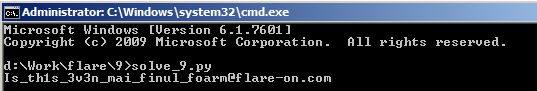This entry was posted in CTF and tagged , , , . Bookmark the permalink.